# Platonic Solids

Platonic Solids

Did you know that the five platonic solids that exist in nature represent the five elements i.e. earth, wind, fire, water, and the universe? Sadly, you won't get an environment-loving superhero coming out of the combination of these five elements.

Check out the interactive simulations to know more about the lesson and try your hand at solving a few interesting practice questions at the end of the page.

But platonic solids are fun to learn, and in this chapter, we will know more about them. In this lesson, you will know more about platonic sold geometry, understand the platonic solid names, and solve some interactive problems.

## Lesson Plan

 1 What are Platonic Solids? 2 Thinking Out of the Box! 3 Important Notes on Platonic Solids 4 Solved Examples on Platonic Solids 5 Interactive Questions on Platonic Solids

## Define Platonic Solids

A platonic solid is a regular, convex polyhedron.

They are named after the ancient Greek philosopher Plato.

A platonic solid has equal and identical faces.

The same number of faces meet at each vertex.

There are 5 regular platonic solids:

2. Cube

3. Octahedron

4. Dodecahedron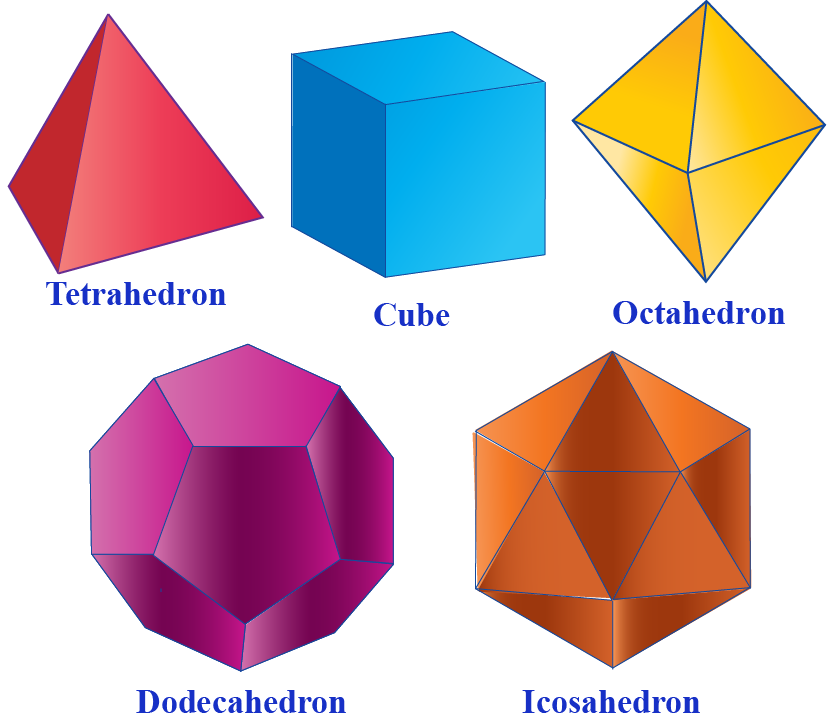## What are the Properties of Platonic Solids?

Following are the properties of a platonic solid:

1. All the faces are regular, convex polyhedron
2. Faces of platonic solid do not intersect except at their edges.
3. The same number of faces meet at each vertex.

## What are the 5 Platonic Solids?

There are 5 regular platonic solids:

1.Tetrahedron:

Tetrahedron
It is made up of equilateral triangles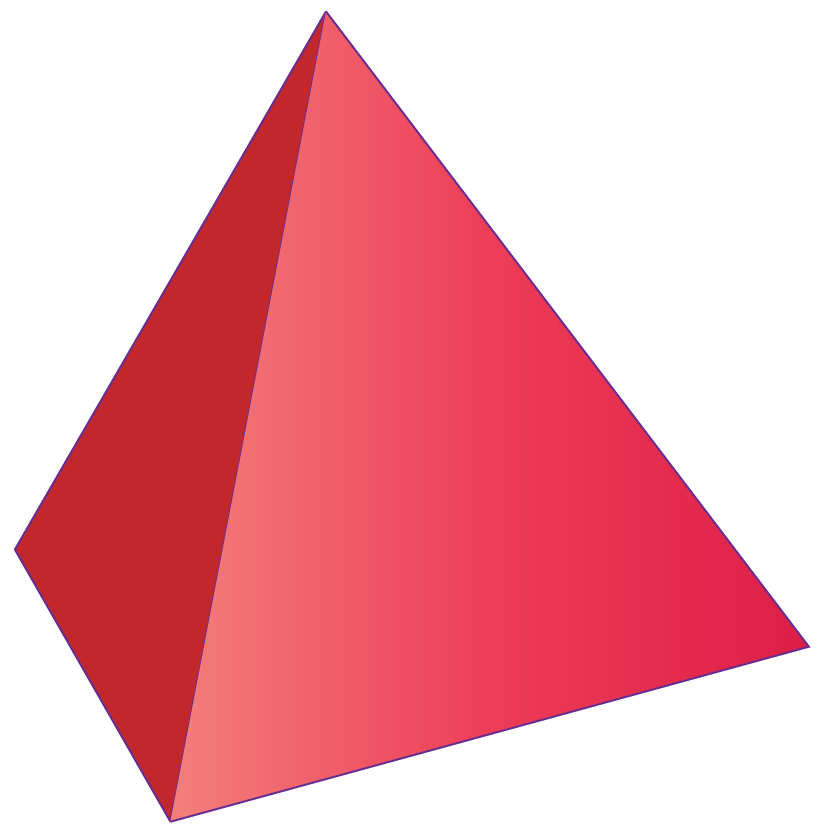Faces 4
Edges 6
Vertices 4
Faces meeting at each vertex 3

2.Cube

Cube
It is made up of square faces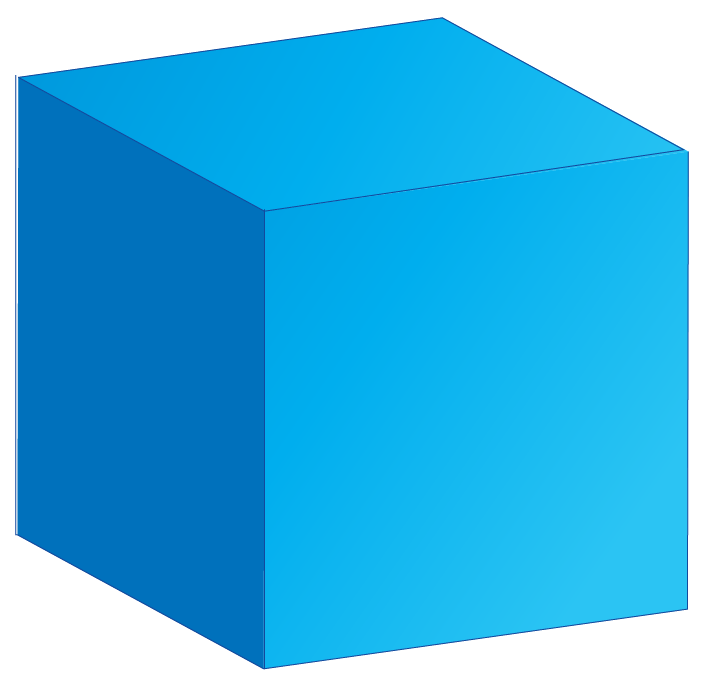Faces 6
Edges 12
Vertices 8
Faces meeting at each vertex 3

3. Octahedron

Octahedron
It is made up of equilateral triangles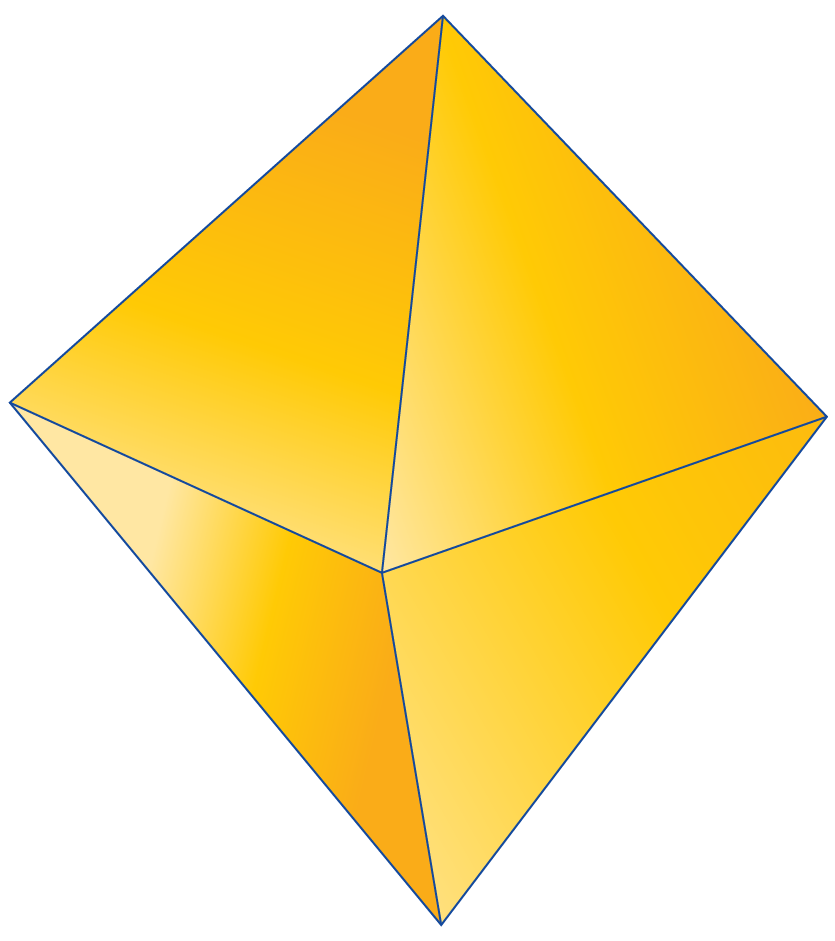Faces 8
Edges 12
Vertices 6
Faces meeting at each vertex 4

4. Dodecahedron

Dodecahedron
It is made up of regular pentagons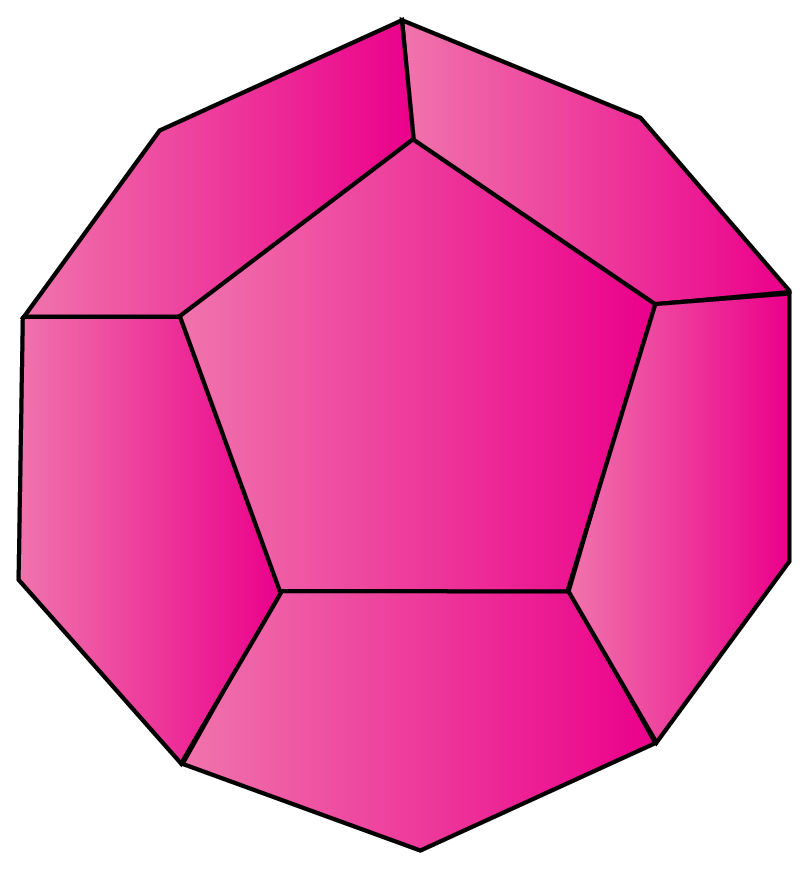Faces 12
Edges 30
Vertices 20
Faces meeting at each vertex 3

5. Icosahedron

Icosahedron
It is made up of equilateral triangles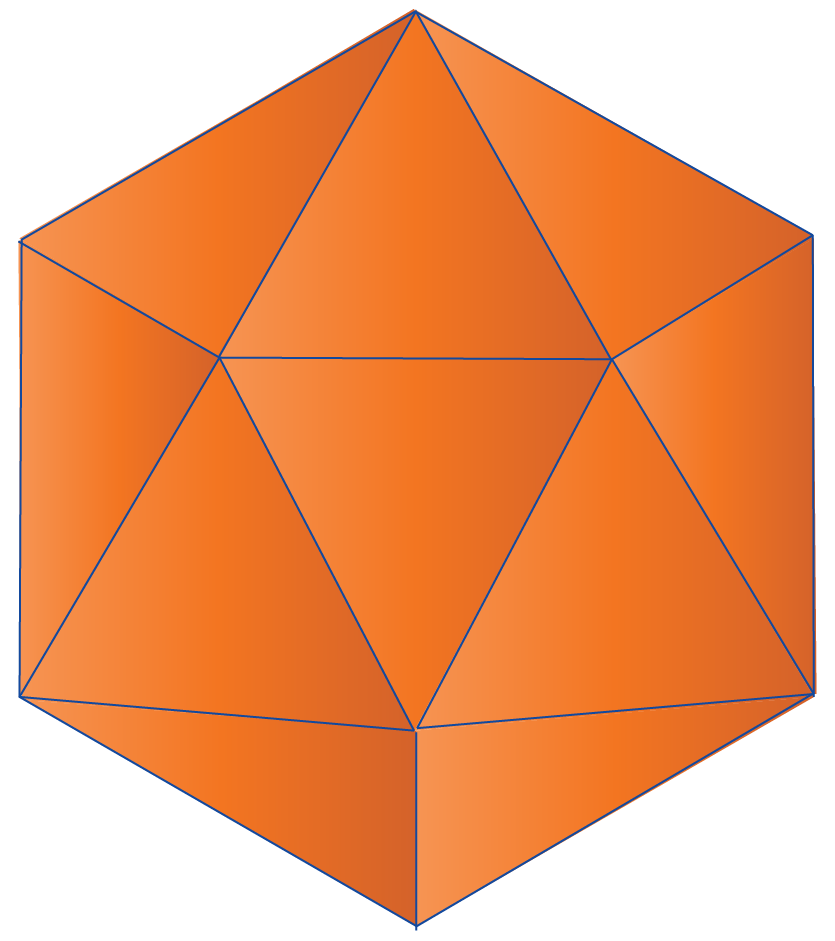Faces 20
Edges 30
Vertices 12
Faces meeting at each vertex 5Think Tank
• Can you figure out how to make all the platonic solids out of the following 2D diagrams?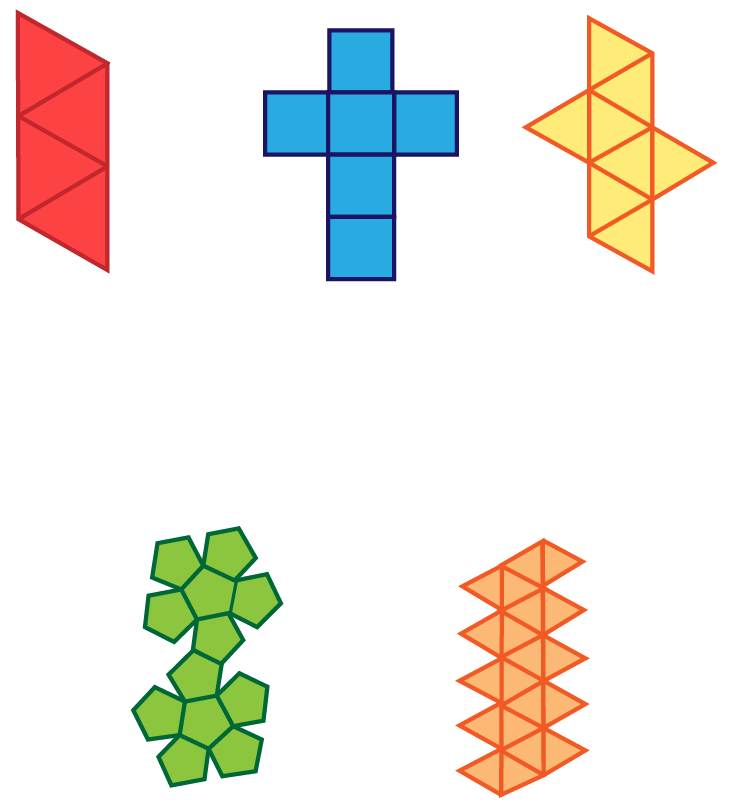Example 3

Demi wants to know the name of the platonic solid shown below. Can you name the platonic solid for her?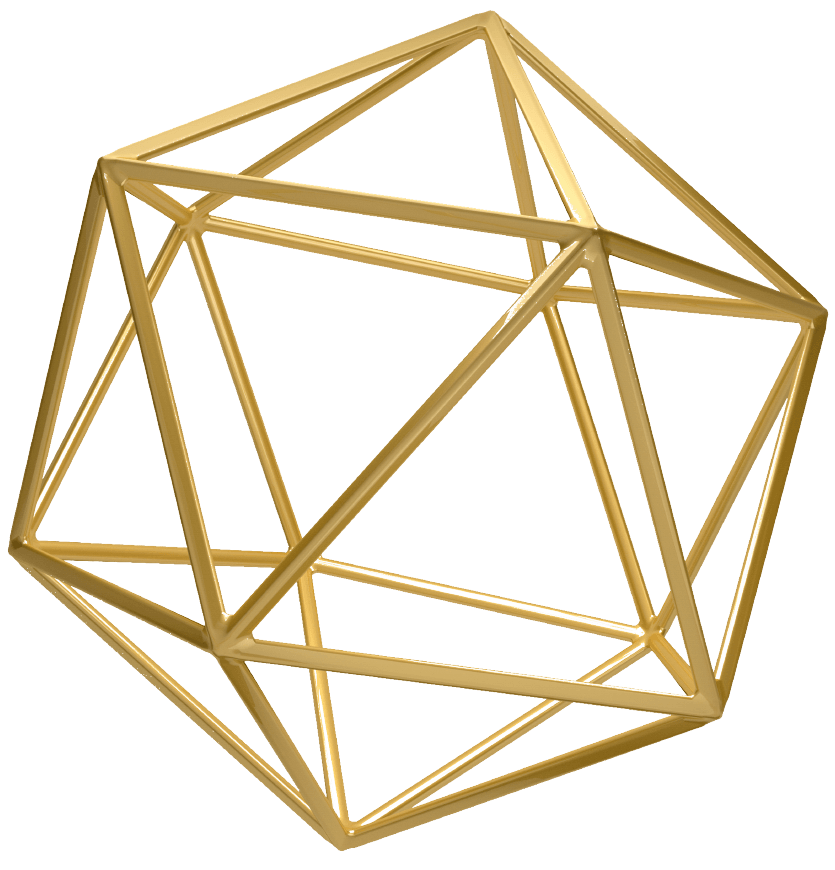Solution
The given solid has 20 triangular faces and 5 triangles are intersecting at each vertex, which is a property of an icosahedron.

Hence the given solid is-

 $$\therefore$$ An Icosahedron
 Example 4

Walter was given a task to complete the following table for platonic solids. Can you help him in completing this table?

Shape Name At each vertex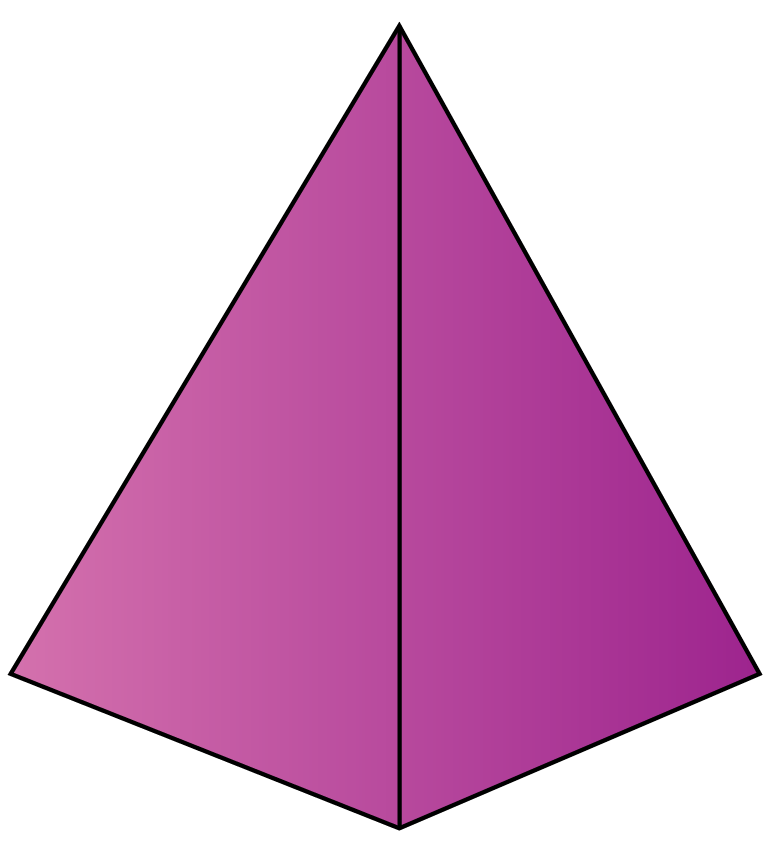______ ______ triangles meet.______ ______ triangles meet.______ ______ pentagons meet.

Solution

Shape Name At each vertexTetrahedron 3 triangles meet.Icosahedron 5 triangles meet.Dodecahedron 3 pentagons meet.
 Example 5

Wendy wants to know the number of faces, vertices, and edges do a dodecahedron has?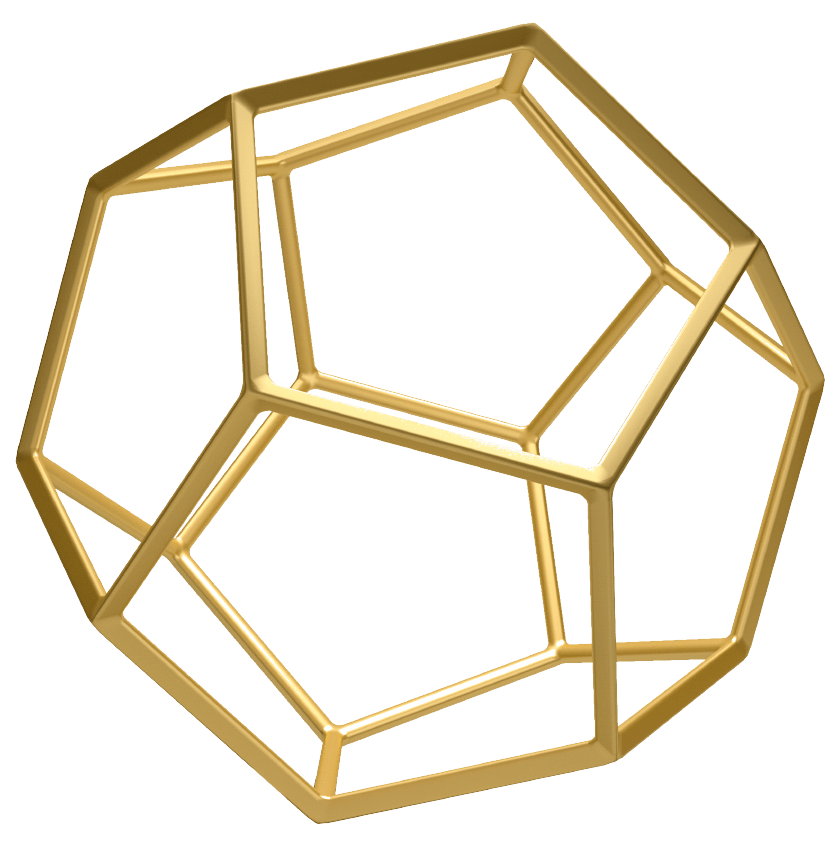Solution

A dodecahedron has:

 $$\therefore$$ 12 faces, 30 edges, and 20 vertices

## Interactive Questions

Here are a few activities for you to practice.

## Let's summarize

The mini-lesson targeted the fascinating concept of platonic solids. The math journey around platonic solids starts with what a student already knows, and goes on to creatively crafting a fresh concept in the young minds. Done in a way that not only it is relatable and easy to grasp, but also will stay with them forever. Here lies the magic with Cuemath.

At Cuemath, our team of math experts is dedicated to making learning fun for our favorite readers, the students!

Through an interactive and engaging learning-teaching-learning approach, the teachers explore all angles of a topic.

Be it worksheets, online classes, doubt sessions, or any other form of relation, it’s the logical thinking and smart learning approach that we, at Cuemath, believe in.

## 1. What do the 5 platonic solids represent?

The cube represents the earth, the octahedron represents the air, the tetrahedron represents the fire, the icosahedron represents the water, and the dodecahedron represents the universe.

## 2.  How many sides platonic solids have?

1.Tetrahedron has 4 sides.

2. Cube has 6 sides.

3. Octahedron has 8 sides.

4. Dodecahedron has 12 sides.

5. Icosahedron has 20 sides.

## 3. Is octahedron a platonic solid?

Octahedron is a regular platonic solid of 8 equilateral sides. So, yes octahedron is a platonic solid.

More Important Topics
Numbers
Algebra
Geometry
Measurement
Money
Data
Trigonometry
Calculus